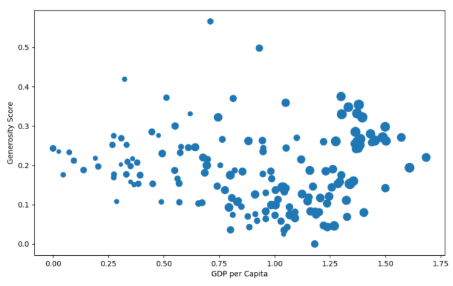# Matplotlib: Change Marker Size in Scatter Plot – A Beginner Example

In matplotlib, we can use plt.scatter() to create a scatter plot. Here is the tutorial:

Matplotlib: Create a Plot Using plt.scatter()

However, we also can change the marker size in the scatter plot. In this tutorial, we will introduce how to do.Look at this example code:

```import matplotlib.pyplot as plt
import pandas as pd

size = df['Score'].to_numpy()
s = [3*s**2 for s in size]

fig, ax = plt.subplots(figsize=(10, 6))
ax.scatter(x = df['GDP per capita'], y = df['Generosity'], s = s)
plt.xlabel("GDP per Capita")
plt.ylabel("Generosity Score")

plt.show()```

As to code:

`ax.scatter(x = df['GDP per capita'], y = df['Generosity'], s = s)`

Here parameter s is the size of marker.

Run this code, you may see this scatter plot: